Ohms Law is definitely one of the most important mathematical relationship between Voltage, Current and Resistance. Ohm’s Law defines the relation between the voltage across a load (resistance) and the current flowing though it. This post will discuss what is Ohms Law, Understanding it through a simple circuit, units of measurement, Ohm’s Law Triangle and when it is not applicable.

## Introduction

The relationship between current, voltage and resistance is known as the Ohm Law. Georg Simon Ohm discovered Ohm’s Law in 1827. This law is used to study electrical circuits.

It explains that the potential difference between two points on a circuit is equal to the product of the current flowing between those two points and the total resistance of all electrical components existing between both points.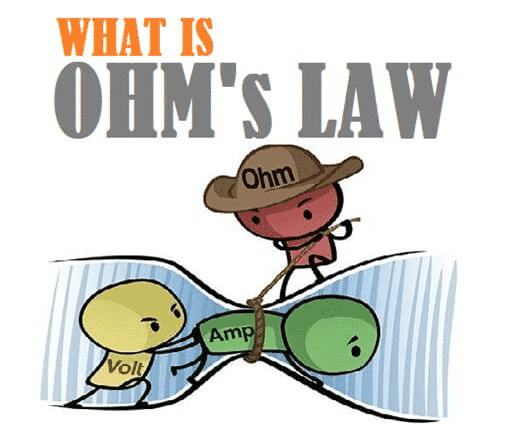Fig. 1 – Introduction to Ohms Law

The greater the voltage of the battery, the higher the current will be. Similarly, there will be less current with greater resistance.

## What is Ohms Law

Ohm’s law says that “The current flowing through two points in a conductor is directly proportional to the potential difference (voltage) across the two points.”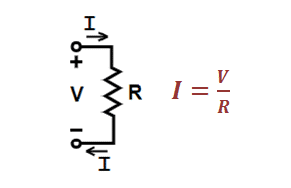Fig. 2 – Parameters of Ohms Law

Where,

• I = Current flowing through the conductor
• V = Voltage measured across the conductor
• R = Resistance of the conductor calculated. R in this relation is constant and independent of the current.

## Units of Measurements in Ohms Law

The factors that require measurements in Ohms Law are Current, Resistance and Voltage. Their Symbol, unit of measurement and unit abbreviation is appended below: –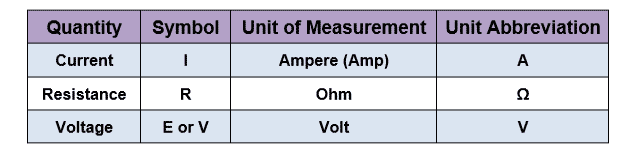Fig. 3 – Units of Measurements in Ohms Law

## Understanding Ohms Law Through Simple Circuit

Ohm’s Law is a simple and useful tool for analyzing electric circuits. It is used often in the study of electricity and electronics.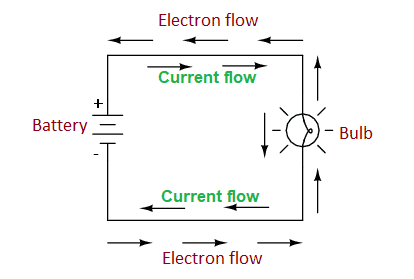Fig. 4 – Understanding Ohms Law Through Simple Circuit

In the above circuit, there is only one source of voltage (Battery) and only one source of resistance to current (Bulb). The identification of any two quantities makes the application of Ohms Law very easy. There are three quantities (factors) in Ohm’s Law. If the values of any two quantities in a circuit is known to you, then you can easily find the third one using Ohm’s Law.

To understand it better, Let us take an example. In the below circuit, the voltage and resistance values are given. We need to find the value of current.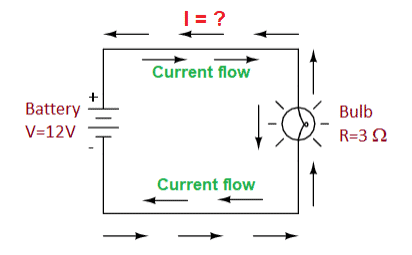Fig. 5 – Applying Ohms Law to the Circuit

As we already know the Ohm’s Law formula i.e. :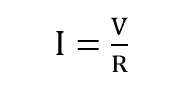Putting the values of Voltage and Resistance from the above circuit, we get: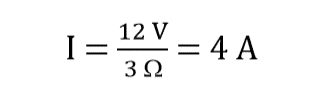## Ohms Law Triangle

Ohm’s Law Triangle is an easy way to remember the Voltage, Current and Resistance relationship. It serves as a trick to find any one of the three quantities, given the other two are known.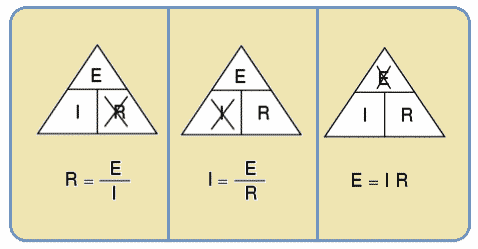Fig. 6 – Ohms Law Triangle

In this, the quantities E, I, and R are arranged in a triangle as shown in the figure above. The voltage (E) is at the top and the other two quantities i.e. Current (I) and resistance (R) are arranged below side by side horizontally. The split between the top and bottom sections indicates division and the line dividing the left and right sections indicates multiplication.

## When is Ohms Law not Applicable

Ohm’s law is an empirical law rather than being a fundamental law. Empirical laws are that laws which are stated based on certain observations. The main assumption in this law is that the total resistance is constant. Though this law works most of the time, it is not applicable for those conductors/ components which are non-ohmic in nature.

Non-Ohmic conductor/ components are those whose resistance changes with temperature. They are also known as Non-Linear Elements. Ohm’s Law is not applicable to all those electrical/ electronic devices which contain any of the following components in their circuitry:

• Tungsten Filament (in Incandescent Bulbs)
• Vacuum Tubes
• Semiconductors
• Transistors
• Liquid Electrolytes
• Thermistors
• Iron Core Inductors
• Transformers
```Also Read:
Power Factor - Power Triangle, Types, PFC, Applications, Advantages
What is Digital Voltmeter - How it Works, Types, Applications, Advantages
Voltmeter - Working Principle, Voltage Sensitivity, Types and Applications
How to Make Simple Inverter at Home - Step by Step```